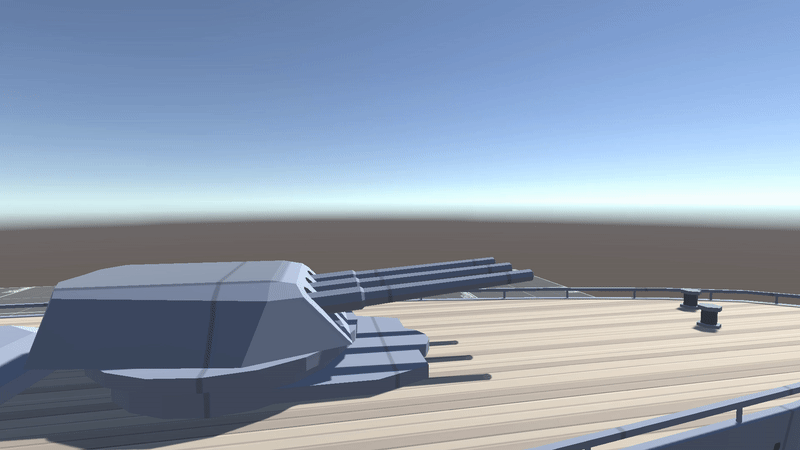# How to Use Quaternion in Unity?

Quaternions are an extension of complex numbers and can be used to represent 3D rotations, spatial translations, and scaling. Invented by Sir William Rowan Hamilton in 1843, these numbers are not easy to understand intuitively. With all honesty, I still don't fully know how it works.

However, in Unity, we can handle the rotation without having to understand them fully. Now, Let’s look at some useful things we can do with Unity Quaternion API

## 1. Slerp

`public static Quaternion Slerp(Quaternion a, Quaternion b, float t);`

Slerp is a spherical interpolation between quaternions `a` and `b` by the ratio of `t`. The parameter `t` is clamped in between [0, 1].

## 2. LookRotation

`public static Quaternion LookRotation(Vector3 forward, Vector3 upwards = Vector3.up);`

Creates a rotation with the specified direction to look in and the vector that defines in which direction up is.

• `forward`: direction to look in
• `upwards` : vector that defines in which direction up is

## 3. Quaternion.Euler

`public static Quaternion Euler(float x, float y, float z);`

Returns a rotation that rotates z degrees around the z-axis, x degrees around the x-axis, and y degrees around the y-axis; applied in that order. We can set a new rotation to a game object with this method.Turret barrels are rotating on their x-axis between 0 & 30 degrees using Quaternion.Euler method.

## 4. Quaternion.RotateTowards

`public static Quaternion RotateTowards(Quaternion from, Quaternion to, float maxDegreesDelta);`

Creates a rotation that rotates from one direction to another direction `maxDegreesDelta` as an angular step towards the target.

`Vector3 rotation = Quaternion.Euler(0,_angle,0) * _rotatingObject.right * _radius;`